## 4.4  Integrable Systems

Islands appear near commensurabilities, and commensurabilities are present even in integrable systems. In integrable systems an infinite number of periodic orbits are associated with each commensurability, but upon perturbation only a finite number of periodic orbits survive. How does this happen? First we have to learn more about integrable systems.

If an n-degree-of-freedom system has n independent conserved quantities then the solution of the problem can be reduced to quadratures. Such a system is called integrable. Typically, the phase space of integrable systems is divided into regions of qualitatively different behavior. For example, the motion of a pendulum is reducible to quadratures and has three distinct types of solutions: the oscillating solutions and the clockwise and counterclockwise circulating solutions. The different regions of the pendulum phase space are separated by the trajectories that are asymptotic to the unstable equilibrium. It turns out that for any system that is reducible to quadratures, a set of phase-space coordinates can be chosen for each region of the phase space so that the Hamiltonian describing the motion in that region depends only on the momenta. Furthermore, if the phase space is bounded then the generalized coordinates can be chosen to be angles (that are 2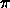-periodic). The configuration space described by n angles is an n-torus. The momenta conjugate to these angles are called actions. Such phase-space coordinates are called action-angle coordinates. We will see later how to reformulate systems in this way. Here we explore the consequences of such a formulation; this formulation is especially useful for finding out what happens as additional effects are added to integrable problems.

#### Orbit types in integrable systems

Suppose we have a time-independent n-degree-of-freedom system that is reducible to quadratures. For each region of phase space there is a local formulation of the system so that the evolution of the system is described by a time-independent Hamiltonian that depends only on the momenta. Suppose further that the coordinates are all angles. Let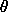be the tuple of angles and J be the tuple of conjugate momenta. The Hamiltonian is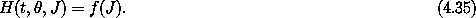Hamilton's equations are simply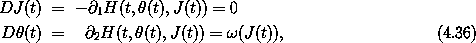where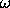(J) = D f(J) is a tuple of frequencies with a component for each degree of freedom. The momenta are all constant because the Hamiltonian does not depend on any of the coordinates. The motion of the coordinate angles is uniform; the rates of change of the angles are the frequencies, which depend only on the constant momenta. Given initial values(t0) and J(t0) at time t0, the solutions are simple: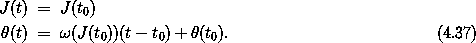Though the solutions are simple, there are a number of distinct orbit types: equilibrium solutions, periodic orbits, and quasiperiodic orbits, depending on the frequency ratios.

If(J) is zero for some J thenand J are both constant, for any. The system is at an equilibrium point.

A solution is periodic if all the coordinates (and momenta) of the system return to their initial values at some later time. Each coordinatei with nonzero frequencyi(J(t0)) is periodic with a period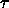i = 2/i(J(t0)). The period of the system must therefore be an integer multiple ki of each of the individual coordinate periodsi. If the system is periodic with some set of integer multiples, then it is also periodic with any common factors divided out. Thus the period of the system is= (ki/d)i where d is the greatest common divisor of the integers ki.

For a system with two degrees of freedom, a solution is periodic if there exists a pair of relatively prime integers k and j such that k0(J(t0)) = j1(J(t0)). The period of the system is= 2j /0(J(t0)) = 2k /1(J(t0)); the frequency is0(J(t0)) / j =1(J(t0)) / k. A periodic motion on the 2-torus is illustrated in figure 4.10.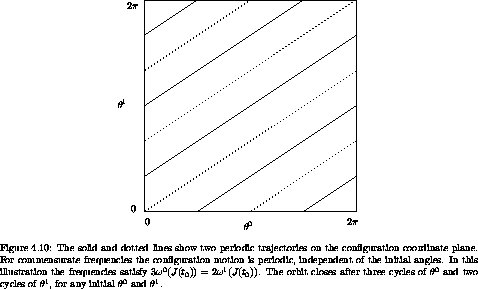If the frequenciesi(J(t0)) satisfy an integer-coefficient relation sumi nii(J(t0)) = 0, we say that the frequencies satisfy a commensurability. If there is no commensurability for any nonzero integer coefficients, we say that the frequencies are linearly independent (with respect to the integers) and the solution is quasiperiodic. One can prove that for n incommensurate frequencies all solutions come arbitrarily close to every point in the configuration space.8

For a system with two degrees of freedom the solutions in a region described by a particular set of action-angle variables are either equilibrium solutions, periodic solutions, or quasiperiodic solutions.9 For systems with more than two degrees of freedom there are trajectories that are neither periodic nor quasiperiodic with n frequencies. These are quasiperiodic with fewer frequencies and dense over a corresponding lower-dimensional torus.

#### Surfaces of section for integrable systems

As we have seen, in action-angle coordinates the angles move with constant angular frequencies, and the momenta are constant. Thus surfaces of section in action-angle coordinates are particularly simple. We can make surfaces of section for time-independent two-degree-of-freedom systems or one-degree-of-freedom systems with periodic drive. In the latter case, one of the angles in the action-angle system is the phase of the drive. We make surfaces of section by accumulating points in one pair of canonical coordinates as the other coordinate goes through some particular value, such as zero. If we plot the section points with the angle coordinate on the abscissa and the conjugate momentum on the ordinate then the section points for all trajectories lie on horizontal lines, as illustrated in figure 4.11.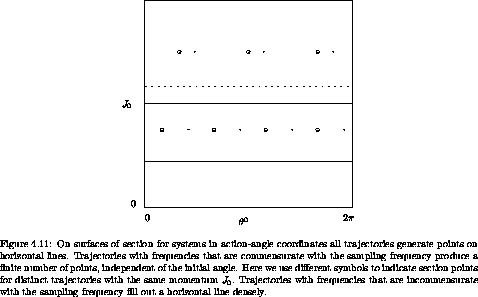For definiteness, let the plane of the surface of section be the (0, J0) plane, and the section condition be1 = 0. The other momentum J1 is chosen so that all the trajectories have the same energy. The momenta are all constant, so for a given trajectory all points that are generated are constrained to a line of constant J0.

The time between section points is the period of1: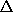t = 2/1(J(t0)) because a section point is generated for every cycle of1. The angle between successive points on the section is0(J(t0))t =0(J(t0)) 2/1(J(t0)) = 2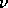(J(t0)), where(J) =0(J) /1(J) is called the rotation number of the trajectory. Let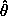(i) and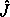(i) be the ith point (i is an integer) in a sequence of points on the surface of section generated by a solution trajectory: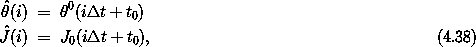where the system is assumed to be on the section at t = t0. Along a trajectory, the map from one section point ((i),(i) ) to the next ((i + 1),(i + 1) ) is of the form:10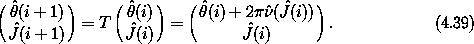As a function of the action on the section, the rotation number is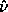((0)) =((0), J1(t0)), where J1(t0) has the value required to be on the section, as for example by giving the correct energy. If the rotation number functionis strictly monotonic in the action coordinate on the section then the map is called a twist map.11

On a surface of section the different types of orbits generate different patterns. If the orbit is an equilibrium solution then the initial point on the surface of section is a fixed point. The system just stays there.

If the two frequencies are commensurate, then the trajectory is periodic and only a finite number of points are generated on the surface of section. Both of the periodic solutions illustrated in figure 4.10 generate two points on the surface of section defined by1 = 0. If the frequencies are commensurate they satisfy a relation of the form k0(J(t0)) = j1(J(t0)), where J(t0) = ((0), J1(t0)) is the initial and constant value of the momentum tuple. The motion is periodic with frequency0(J(t0))/j =1(J(t0))/k, so the period is 2j/0(J(t0)) = 2k/1(J(t0)). Thus this periodic orbit generates k points on this surface of section. For trajectories with commensurate frequencies the rotation number is rational:((0)) =((0), J1(t0)) = j/k. The coordinate1 makes k cycles while the coordinate0 makes j cycles (figure 4.10 shows a system with a rotation number of 2/3). The frequencies depend on the momenta but not on the coordinates, so the motion is periodic with the same period and rotation number for all initial angles given these momenta. Thus there is a continuous family of periodic orbits with different initial angles.

If the two frequencies are incommensurate, then the 2-torus is filled densely. Thus the line on which the section points are generated is filled densely. Again, this is the case for any initial coordinates, because the frequencies depend only on the momenta. There are infinitely many such orbits that are distinct for a given set of frequencies.12

8 Motion with n incommensurate frequencies is dense on the n-torus. Furthermore, such motion is ergodic on the n-torus. This means that time averages of time-independent phase-space functions computed along trajectories are equal to the phase-space average of the same function over the torus.

9 For time-independent systems with two degrees of freedom the boundary between regions described by different action-angle coordinates has asymptotic solutions and unstable periodic orbits or equilibrium points. The solutions on the boundary are not described by the action-angle Hamiltonian.

10 The coordinate(i) is an angle. It can be brought to a standard interval such as 0 to 2.

11 Actually, for a map to be a twist map we require | D(J) | > K > 0 over some interval of J.

12 The section points for any particular orbit are countable and dense, but they have zero measure on the line.